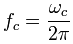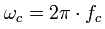Deutsche VersionFrequency response and equalization (EQ filter) ●

Preemphasis and deemphasis - Frequency and angular frequency

Conversion: time constant τ to cut-off frequency  fc and
cut-off frequency (corner frequency) fc to time constant τ

cut-off frequency = corner frequency = break frequency

 Time constant µs | Cut-off frequency Hz | ↓ | ↓ | Cut-off frequency Hz | Time constant µs |
 Fill in the box above and click at the appropriate 'calculate' below. The Greek letter for the time constant is tau = τ and 1 microsecond is 10−6 seconds.

 Time constant τ  in µs Cut-off  frequency fc  in Hz Equalization  emphasis 7958 20 • (RIAA) 3183 50 • RIAA, NAB 1592 100 318 500 • RIAA 200 796 140 1137 120 1326 MC 100 1592 90 1768 MC 75 2122 • RIAA, FM 70 2274 50 3183 NAB, PCM, FM 35 4547 DIN 25 6366 17.5 9095 AES 15 10610 PCM 12.5 12732 10 15915

Cut-off frequency: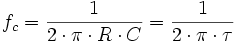Time constant: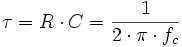Conditional equations:
Cut-off frequency fc in Hz = 159155 / τ in µs
Time constant τ in µs = 159155 / fc in Hz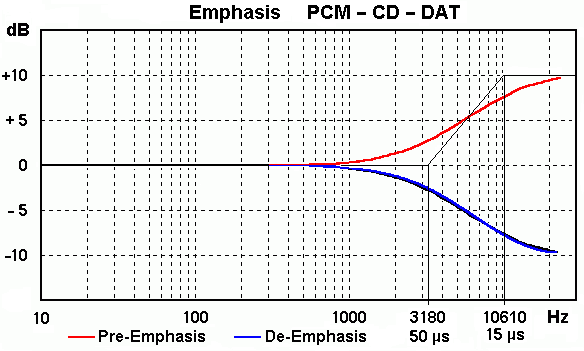The possible pre-emphasis/de-emphasis of a PCM recording for DAT or CD (15/50 µs EIAJ DAT/CD).

Cut-off frequency:Time constant:Conditional equations:
Cut-off frequency fc in Hz = 159155 / τ in µs
Time constant τ in µs = 159155 / fc in HzAt the cut-off frequency fc of a drop, the voltage V is always fallen to the value of 1/√2 and the voltage level is damped to 20 × log(1/√2) = (−)3,0103 dB.

Time constant and cut-off frequency

AME - Ampex Master Equalization Curve
Pre-Emphasis and De-Emphasis or preemphasis and deemphasis

 There was also an "Ampex Master Equalization" around the year 1958, called AME. It was based on modifying the NAB response with an auditory (hearing) curve, which resulted in a recorded flux that had a "hump" (re the NAB EQ) from about +3 dB at 630 Hz to +8 dB at 2 kHz, back to +3 dB at 5 kHz, and down to −12 dB at 16 kHz; see the AME curve: http://home.comcast.net/~mrltapes/pubame.pdf http://www.richardhess.com/tape/ame_equalizer_20040412.pdf

 In USA FM radio has a time constant of 75 µs, but also in Korea, Taiwan, Thailand, AFN worldwide and the rest of America. In Europe FM radio has a time constant of 50 µs, but also in Africa, Asia (without Korea, Taiwan, Thailand), Pacific.

Record RIAA cutting equalization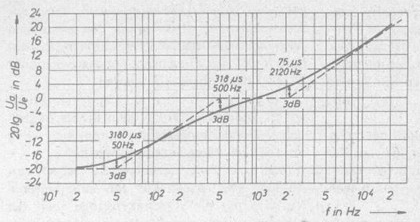Conversion

 To use the calculator, simply enter a value. The calculator works in both directions of the ↔ sign.

 Cut-off frequency fc  Hz ↔ Time constant τ   µs1 microsecond = 10−6 second = 0.000001 second

Time constant τ = R × C            R = τ / C            C = τ / R

Time constant in seconds = Resistance in ohms × Capacitance in Farad

Please enter two values, the third value will be calculated.

 Time constant τ microseconds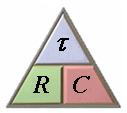Resistance R ohms Capacitance C microfarad
 Corner angular frequency ωc = Angular frequency ωc The corner angular frequency ωc is developed from the corner frequency fc: ωc = 2 π × fc Corner frequency fc = Cut-off frequency fc For systems that correspond to a differential equation of first grades the cutoff point is the intersection of the horizontal asymptote with the asymptote of the falling branch of the Bode diagram. At this point, the level is −3 dB and the phase shift is 45°. This means that the amplitude drop of the output value reached 30% of the input size. At the same time the time constant τ of such system is: τ = 1 / ωc = 1 / 2 π × fc

Cut-off frequency to corner angular frequency and vice versa

 To use the calculator, simply enter a value. The calculator works in both directions of the ↔ sign.

 rad = radiant Cut-off frequency fc  Hz ↔ Corner angular frequency ωc  rad/s